# Non Verbal Reasoning - Dot Situation

### Exercise :: Dot Situation - Section 2

From amongst the figures marked (1), (2), (3) and (4), select the figure which satisfies the same conditions of placement of the dots as in figure (X).

1.

Select the figure which satisfies the same conditions of placement of the dots as in Figure-X.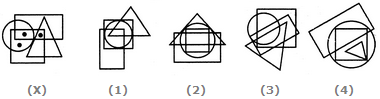A. 1 B. 2 C. 3 D. 4

Explanation:

In fig. (X), one of the dots lies in the region common to the circle and the square only, another dot lies in the region common to the circle, the square and the rectangle only Dot and the third dot lies in the region common to the square, the triangle and the rectangle only. In each of the figures (1), (2) and (4), there is no region common to the square, the triangle and the rectangle only. Only fig. (3) consists of all the three types of regions.

2.

Select the figure which satisfies the same conditions of placement of the dots as in Figure-X.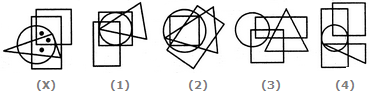A. 1 B. 2 C. 3 D. 4

Explanation:

In fig. (X), one of the dots lies in the region common to the circle, the square and the rectangle only, another dot lies in the region common to all the four elements - the circle, the square, the triangle and the rectangle and the third dot lies in the region common to the circle, the triangle and the rectangle only. In fig. (1), there is no region common to the circle, the triangle and the rectangle only. In each of the figures (3) and (4) there is no region common to all the four elements - the circle, the square, the triangle and the rectangle. Only fig. (2) consists of all the three types of regions.

3.

Select the figure which satisfies the same conditions of placement of the dots as in Figure-X.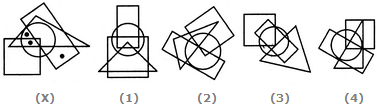A. 1 B. 2 C. 3 D. 4

Explanation:

In fig. (X), one of the dots lies in the region common to the circle and the triangle only, another dot lies in the region common to the circle, the square and the triangle only and the third dot lies in the rectangle alone. In figures (1) and (4) there is no region common to the circle and the triangle only. In fig. (3) there is no region which lies in the rectangle alone. Only fig. (2) contains all the three types of regions.

4.

Select the figure which satisfies the same conditions of placement of the dots as in Figure-X.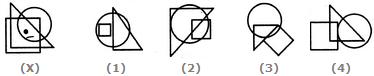A. 1 B. 2 C. 3 D. 4

Explanation:

In fig. (X), the dot lies in the region common to all the three figures - square, triangle and circle. Out of the four alternatives, only fig. (2) contains a region common to all the three figures. Hence, fig. (2) is the answer.

5.

Select the figure which satisfies the same conditions of placement of the dots as in Figure-X.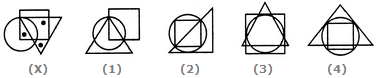A. 1 B. 2 C. 3 D. 4